OK, so suppose we don't have the graph of a function to look at like in the last section...

Can we still find the domain and range?

 Domains: Yes (as long as the algebra doesn't get too hairy... and it won't for us.)
 Ranges: Not really (you usually need the picture -- unless it's something really basic.)

So, we'll just be doing domains on these -- which is really where the action is anyway.

Asking for the domain of a function is the same as asking

"What are all the possible x guys
that I can stick into this thing?"

Sometimes, what you'll really be looking for is

"Is there anything I CAN'T stick in?"

Check it out:

 Let's find the domain of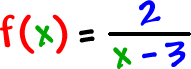Do you see any x guys that would cause a problem here?

 What about?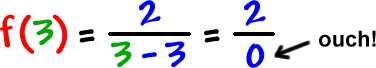So, x = 3 is a bad guy!  Everyone else is OK, though.

The domain is all real numbers except 3.

What would the interval notation be?

When in doubt, graph it on a number line:Do the interval notation in two pieces:

 domain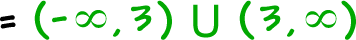Find the domain of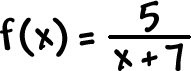Sometimes, you can't find the domain with a quick look.

Check it out:

 Let's find the domain of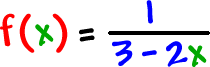Hmm...  It's not so obvious!

BUT, we are still looking for the same thing: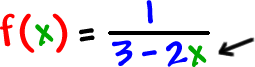The bad x that makes the denominator 0!

How do we find it?  Easy!

Set the denominator = 0 and solve!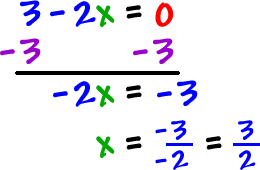The domain is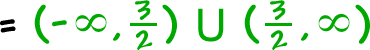TRY IT:

 Find the domain of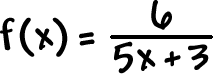*show work!!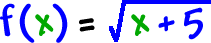Square roots -- what do we know about square roots?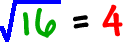...  So, 16 is OK to put in.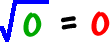...  So, 0 is OK.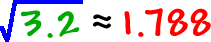...  Yuck!  But, 3.2 is OK.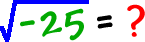...  Nope!  Can't do it!

*We only want real numbers!

No negatives are OK!The inside of a radical cannot be negative if we want real answers only (no i guys).  So, the inside of a radical has to be 0 or a positive number.

 Set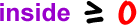and solve it!

Now, let's find the domain ofSo, the domain ofis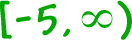.

TRY IT:

 Find the domain of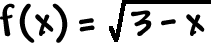. *Show work!!

Here's a messier one:

 Let's find the domain of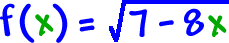Setand solve!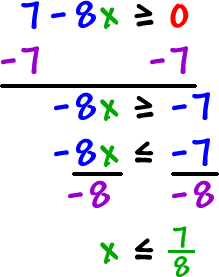The domain is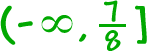.

 Find the domain of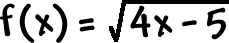.  *Show work!